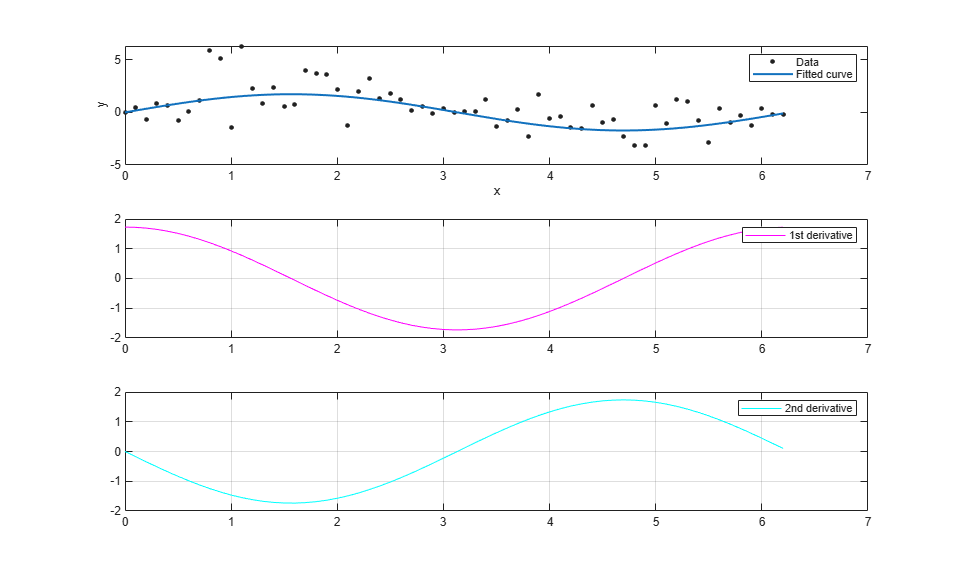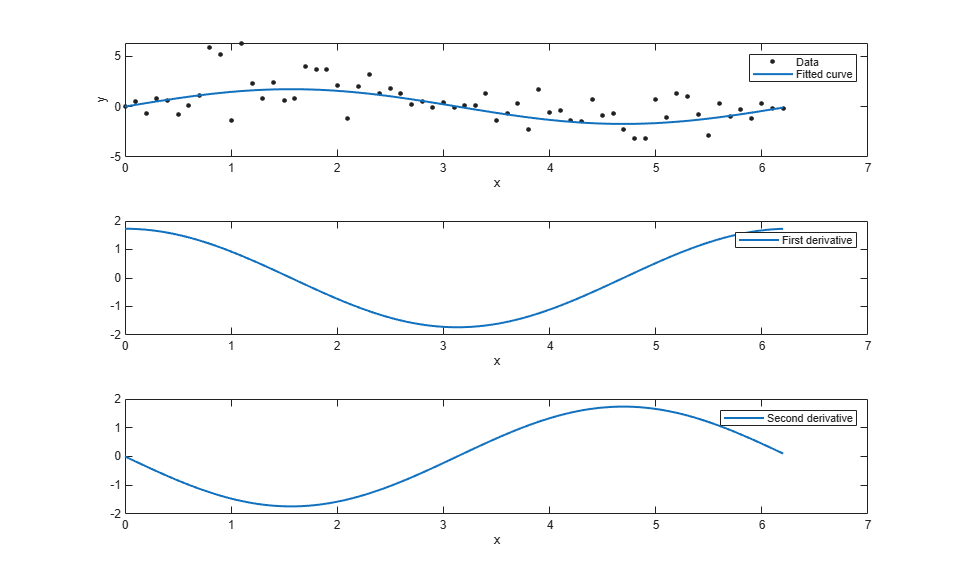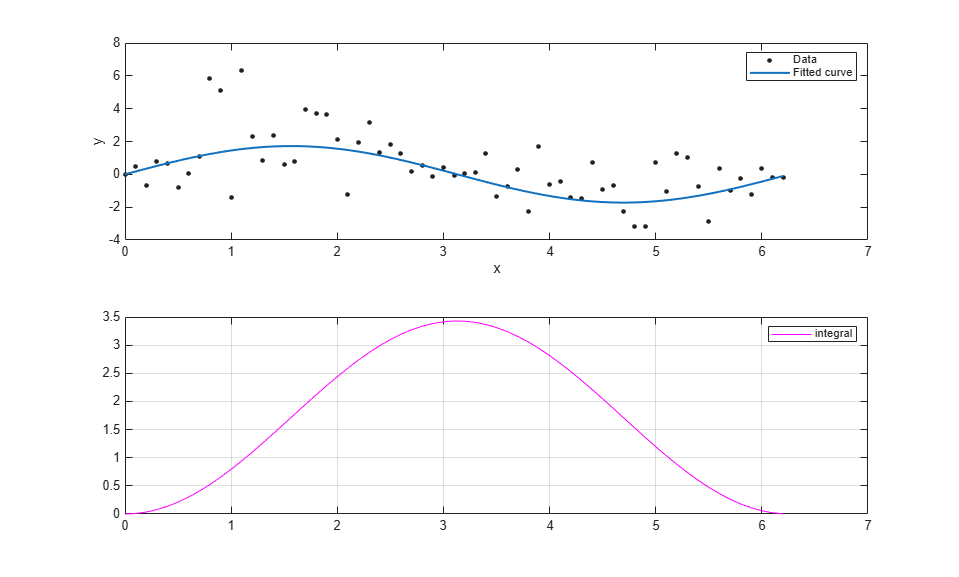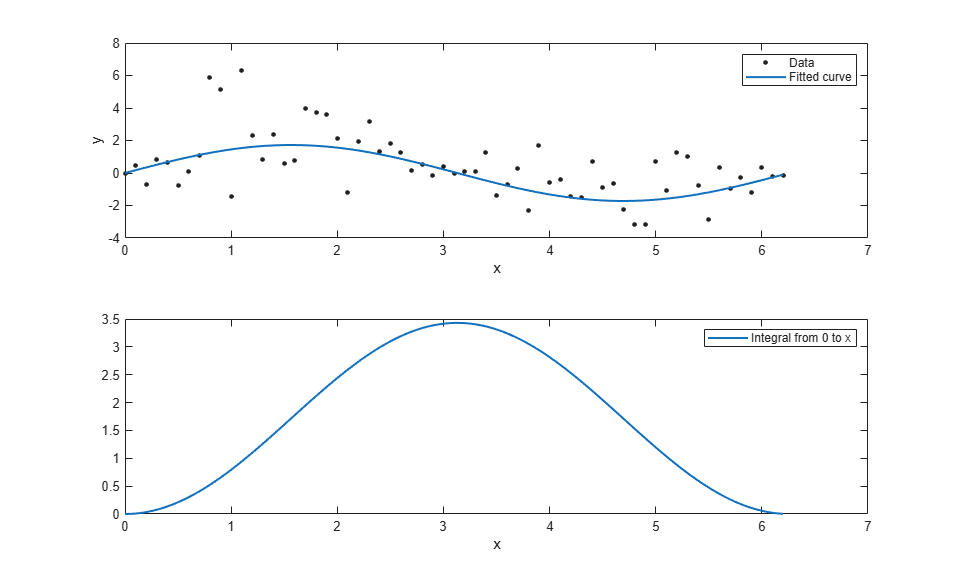Main Content

# Differentiating and Integrating a Fit

This example shows how to find the first and second derivatives of a fit, and the integral of the fit, at the predictor values.

Create a baseline sinusoidal signal:

```xdata = (0:.1:2*pi)'; y0 = sin(xdata); ```

Add noise to the signal:

```noise = 2*y0.*randn(size(y0)); % Response-dependent noise ydata = y0 + noise; ```

Fit the noisy data with a custom sinusoidal model:

```f = fittype('a*sin(b*x)'); fit1 = fit(xdata,ydata,f,'StartPoint',[1 1]); ```

Find the derivatives of the fit at the predictors:

```[d1,d2] = differentiate(fit1,xdata); ```

Plot the data, the fit, and the derivatives:

```subplot(3,1,1) plot(fit1,xdata,ydata) % cfit plot method subplot(3,1,2) plot(xdata,d1,'m') % double plot method grid on legend('1st derivative') subplot(3,1,3) plot(xdata,d2,'c') % double plot method grid on legend('2nd derivative') ```Note that derivatives can also be computed and plotted directly with the cfit plot method, as follows. The plot method, however, does not return data on the derivatives.

```plot(fit1,xdata,ydata,{'fit','deriv1','deriv2'}) ```Find the integral of the fit at the predictors:

```int = integrate(fit1,xdata,0); ```

Plot the data, the fit, and the integral:

```subplot(2,1,1) plot(fit1,xdata,ydata) % cfit plot method subplot(2,1,2) plot(xdata,int,'m') % double plot method grid on legend('integral') ```Note that integrals can also be computed and plotted directly with the cfit plot method, as follows. The plot method, however, does not return data on the integral.

```plot(fit1,xdata,ydata,{'fit','integral'}) ```## Support

#### Machine Learning Challenges: Choosing the Best Classification Model and Avoiding Overfitting

Download white paper